# Hardest colleges to get into in Ohio

Top 10 colleges in Ohio with the lowest acceptance rates
What are the hardest colleges to get into in Ohio? We've got you covered. We've compiled a national college database and have created a list of the hardest universities to get into in Ohio below. These are the hardest 4 year colleges to get into in Ohio, and sometimes some of the hardest colleges to get into in the US. You could even say these are the best colleges in Ohio. We also include each college's average SAT and ACT scores so that you can see where you're most competitive. Read on to find out more.

## Kettering College acceptance rate

Kettering College acceptance rate is 28%.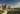The average SAT score for Kettering College is 1065.

• SAT composite: 1065
• SAT math: 565

The average ACT score for Kettering College is 23.

## Case Western Reserve University acceptance rate

Case Western Reserve University acceptance rate is 29.3%.The average SAT score for Case Western Reserve University is 1435.

• SAT composite: 1435
• SAT math: 745

The average ACT score for Case Western Reserve University is 32.

## Denison University acceptance rate

Denison University acceptance rate is 33.9%.The average SAT score for Denison University is 1300.

• SAT composite: 1300
• SAT math: 660

The average ACT score for Denison University is 30.

## Kenyon College acceptance rate

Kenyon College acceptance rate is 35.8%.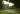The average SAT score for Kenyon College is 1380.

• SAT composite: 1380
• SAT math: 690

The average ACT score for Kenyon College is 31.

## Ohio State University acceptance rate

Ohio State University acceptance rate is 53.7%.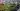The average SAT score for Ohio State University is 1355.

• SAT composite: 1355
• SAT math: 710

The average ACT score for Ohio State University is 30.

## The College of Wooster acceptance rate

The College of Wooster acceptance rate is 56.4%.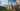The average SAT score for The College of Wooster is 1268.

• SAT composite: 1268
• SAT math: 640

The average ACT score for The College of Wooster is 27.

## Defiance College acceptance rate

Defiance College acceptance rate is 56.5%.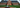The average SAT score for Defiance College is 970.

• SAT composite: 970
• SAT math: 490

The average ACT score for Defiance College is 20.

## Central State University acceptance rate

Central State University acceptance rate is 57.1%.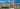The average SAT score for Central State University is 800.

• SAT composite: 800
• SAT math: 390

The average ACT score for Central State University is 15.

## Hiram College acceptance rate

Hiram College acceptance rate is 57.6%.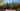The average SAT score for Hiram College is 1068.

• SAT composite: 1068
• SAT math: 530

The average ACT score for Hiram College is 22.

## Mount Saint Joseph University acceptance rate

Mount Saint Joseph University acceptance rate is 60.3%.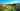The average SAT score for Mount Saint Joseph University is 1060.

• SAT composite: 1060
• SAT math: 520